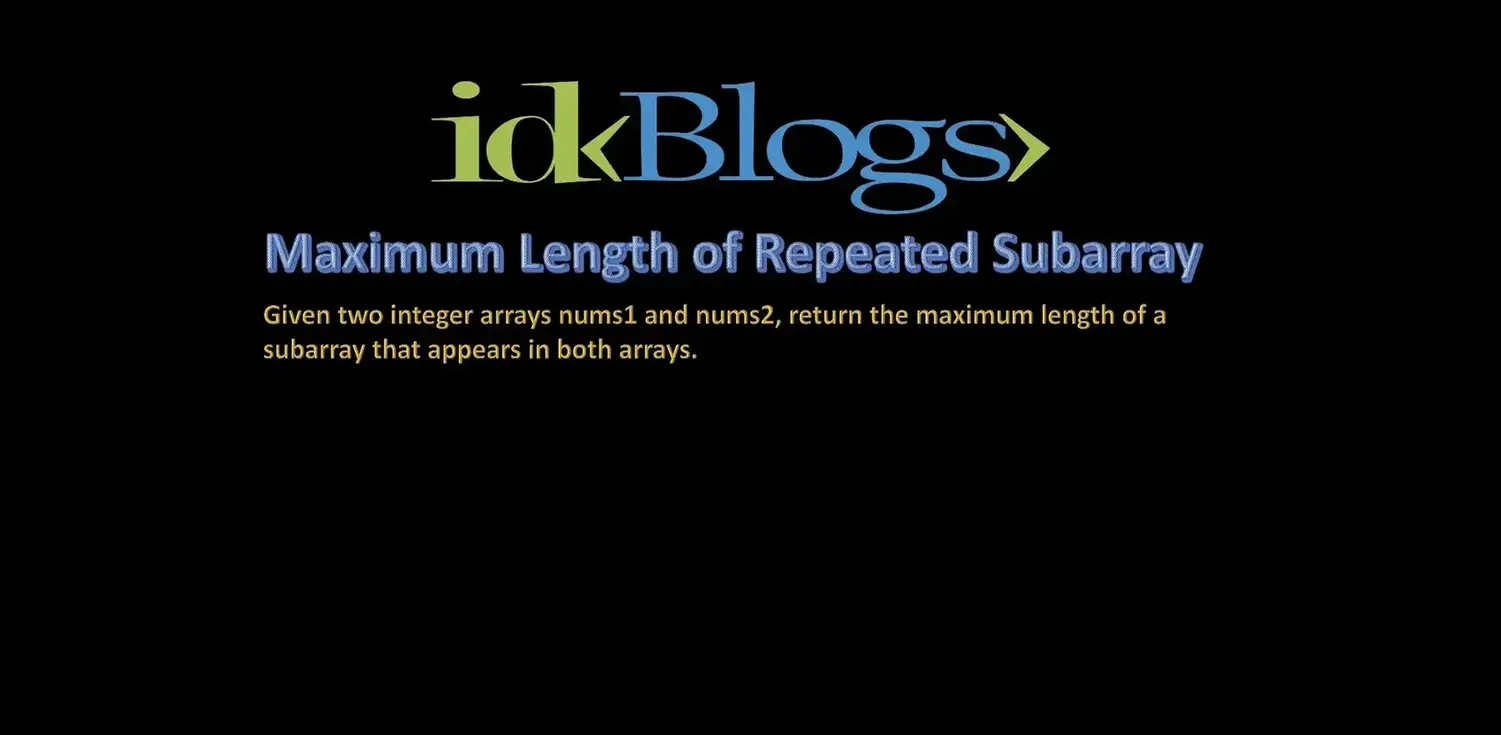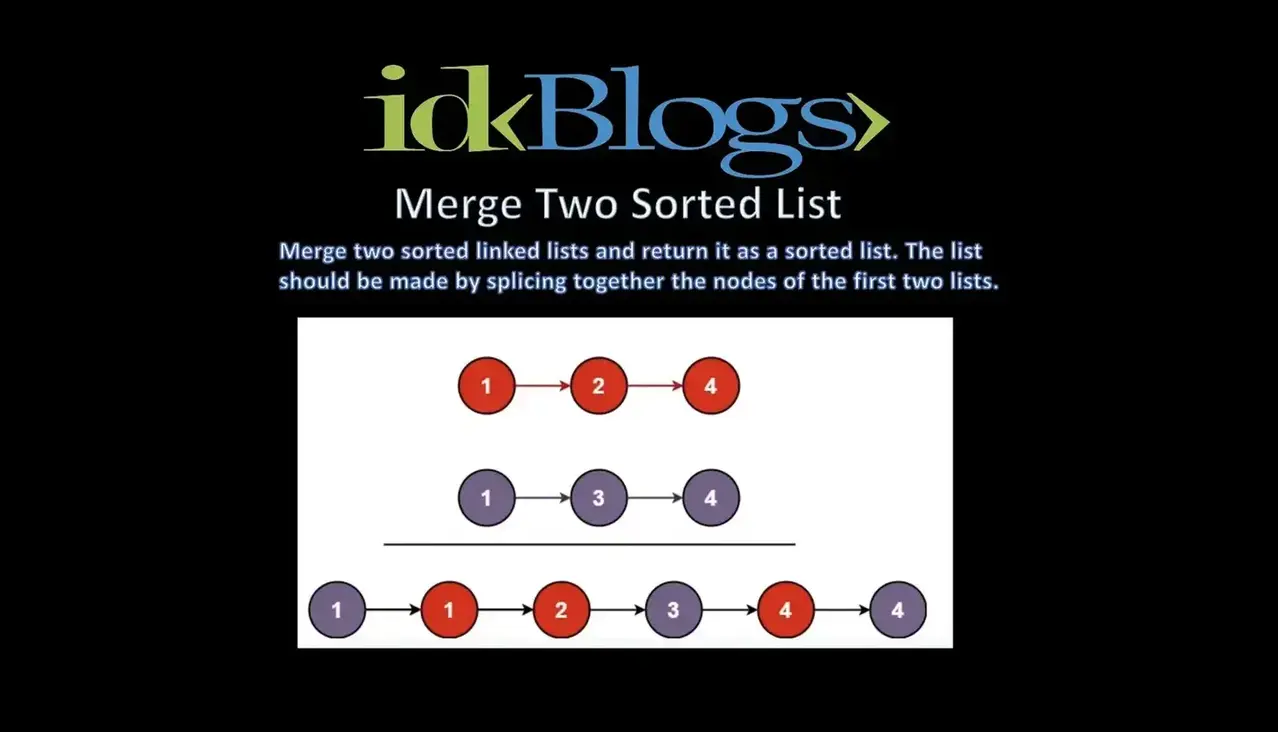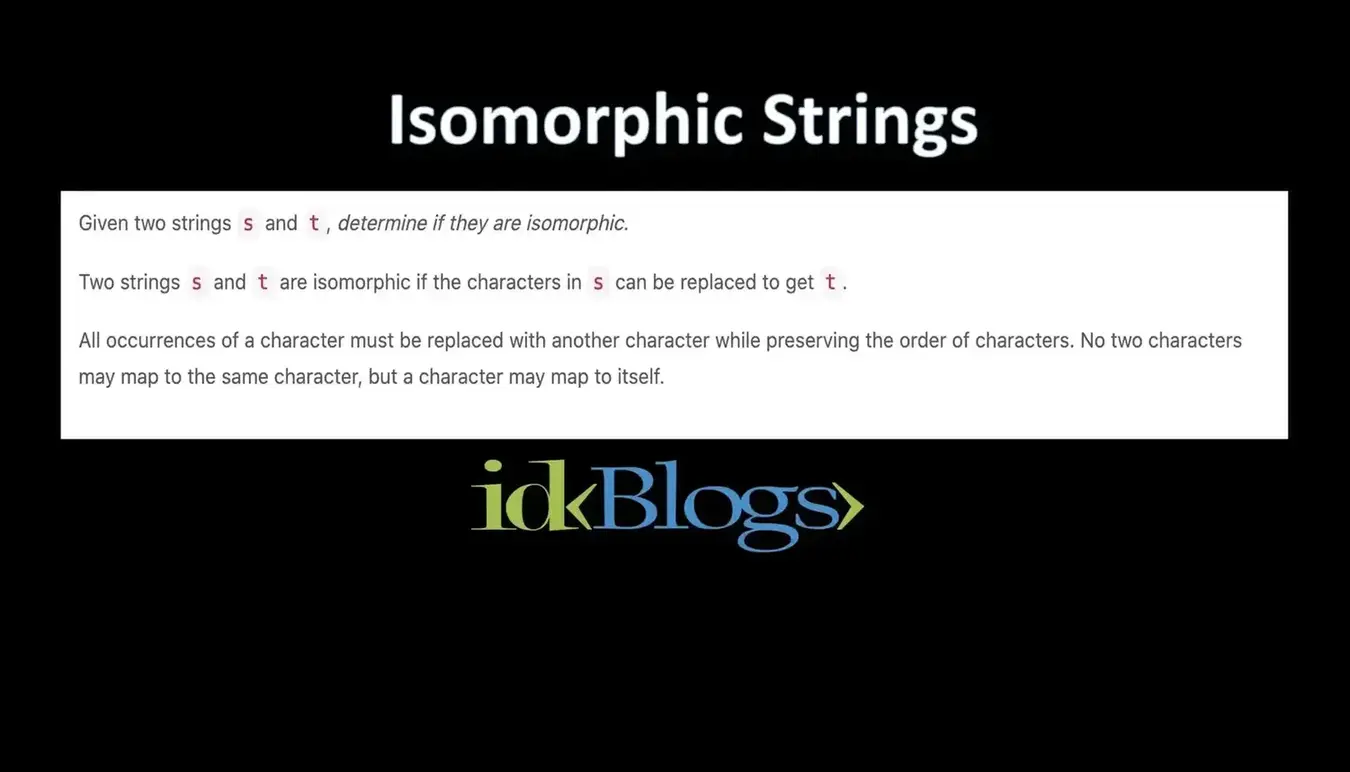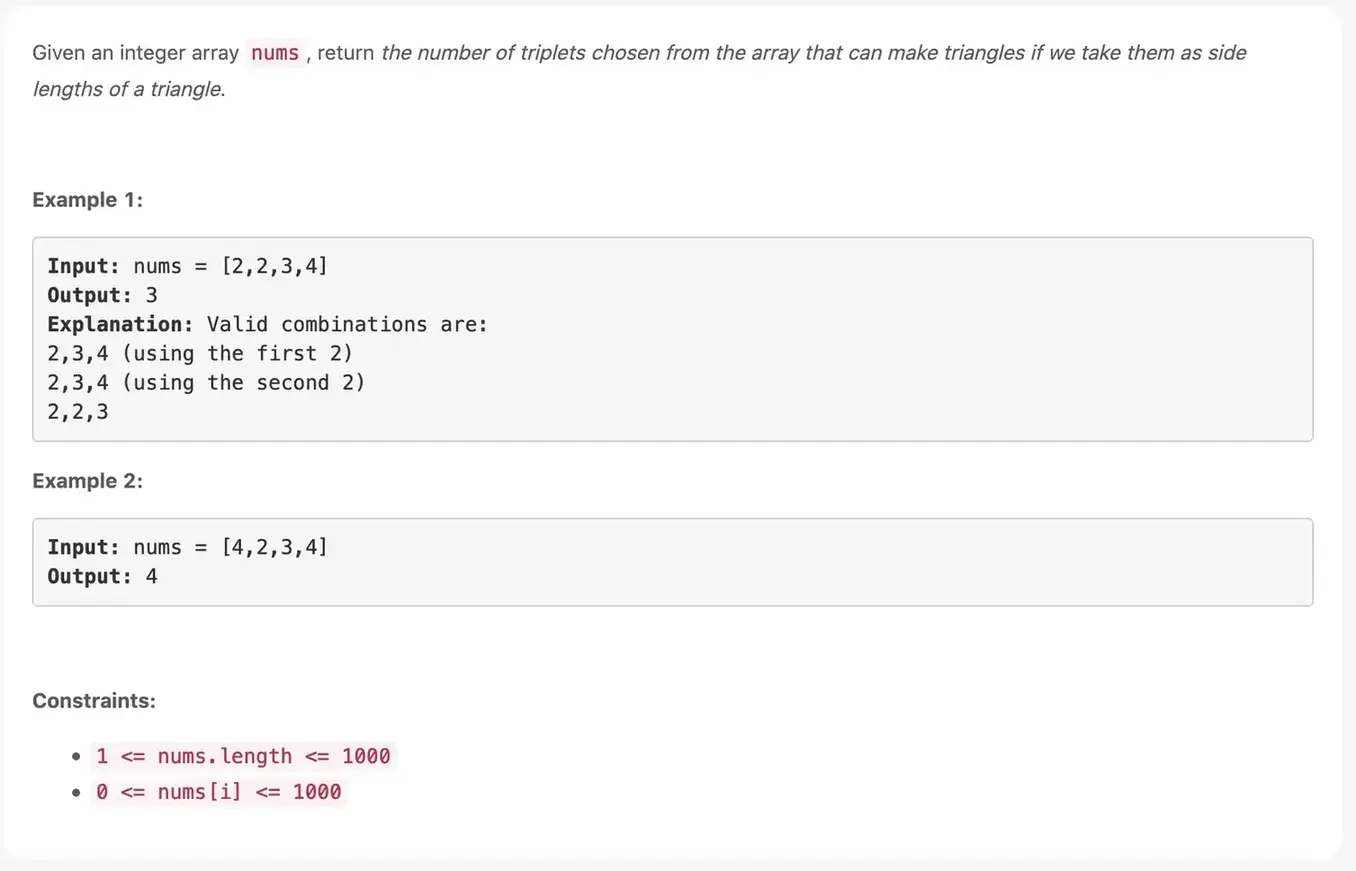# Find Median from Data Stream

### The median is the middle value in an ordered integer list. If the size of the list is even, there is no middle value and the median is the mean of the two middle values.

idkblogs.com      July 11, 2021Shubham Verma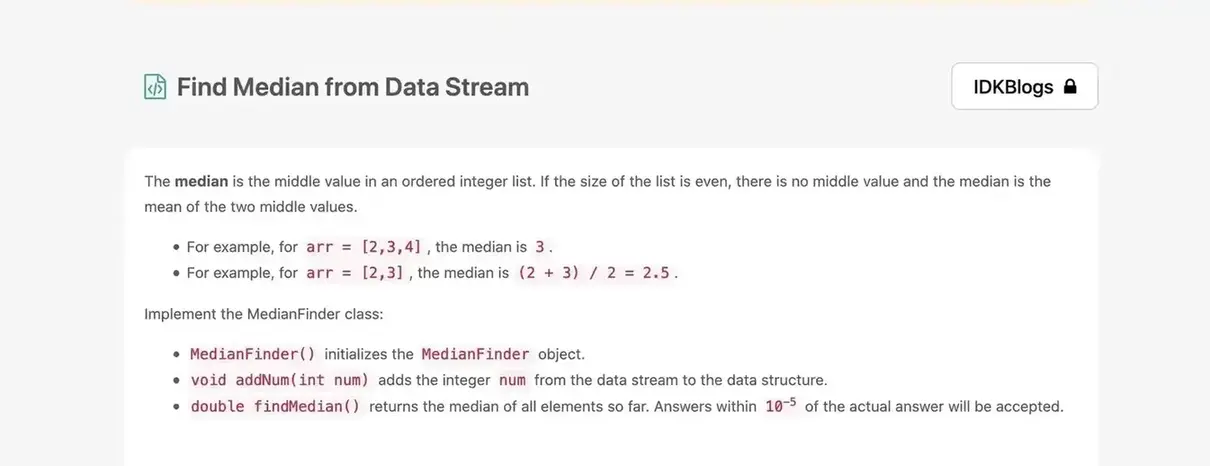# Find Median from Data Stream:

The median is the middle value in an ordered integer list. If the size of the list is even, there is no middle value and the median is the mean of the two middle values.

For example, for arr = [2,3,4], the median is 3.

For example, for arr = [2,3], the median is (2 + 3) / 2 = 2.5.

# Implement the MedianFinder class:

MedianFinder() initializes the MedianFinder object.

void addNum(int num) adds the integer num from the data stream to the data structure.

double findMedian() returns the median of all elements so far. Answers within 10-5 of the actual answer will be accepted.

# Input:

[[], , , [], , []]

# Output:

[null, null, null, 1.5, null, 2.0]

# Explanation:

MedianFinder medianFinder = new MedianFinder();

medianFinder.addNum(2); // arr = [1, 2]

medianFinder.findMedian(); // return 1.5 (i.e., (1 + 2) / 2)

medianFinder.findMedian(); // return 2.0

# Constraints:

-105 <= num <=105

There will be at least one element in the data structure before calling findMedian.

At most 5 * 104 calls will be made to addNum and findMedian.

If all integer numbers from the stream are in the range [0, 100], how would you optimize your solution?
If 99% of all integer numbers from the stream are in the range [0, 100], how would you optimize your solution?

# Solution:

``````                                   /**
* initialize your data structure here.
*/
var MedianFinder = function() {
this.num=[];
this.findMedian;
};

/**
* @param {number} num
* @return {void}
*/
this.num.push(num);
};

/**
* @return {number}
*/
MedianFinder.prototype.findMedian = function() {
let a = this.num;
let n =this.num.length;
// First we sort the array
a.sort();

// check for even case
if (n % 2 !== 0){
return a[parseInt(n / 2)];
}else{
return (a[parseInt((n - 1) / 2)] + a[parseInt(n / 2)]) / 2.0;
}

};

/**
* Your MedianFinder object will be instantiated and called as such:
* var obj = new MedianFinder()
* var param_2 = obj.findMedian()
*/
``````

# Output: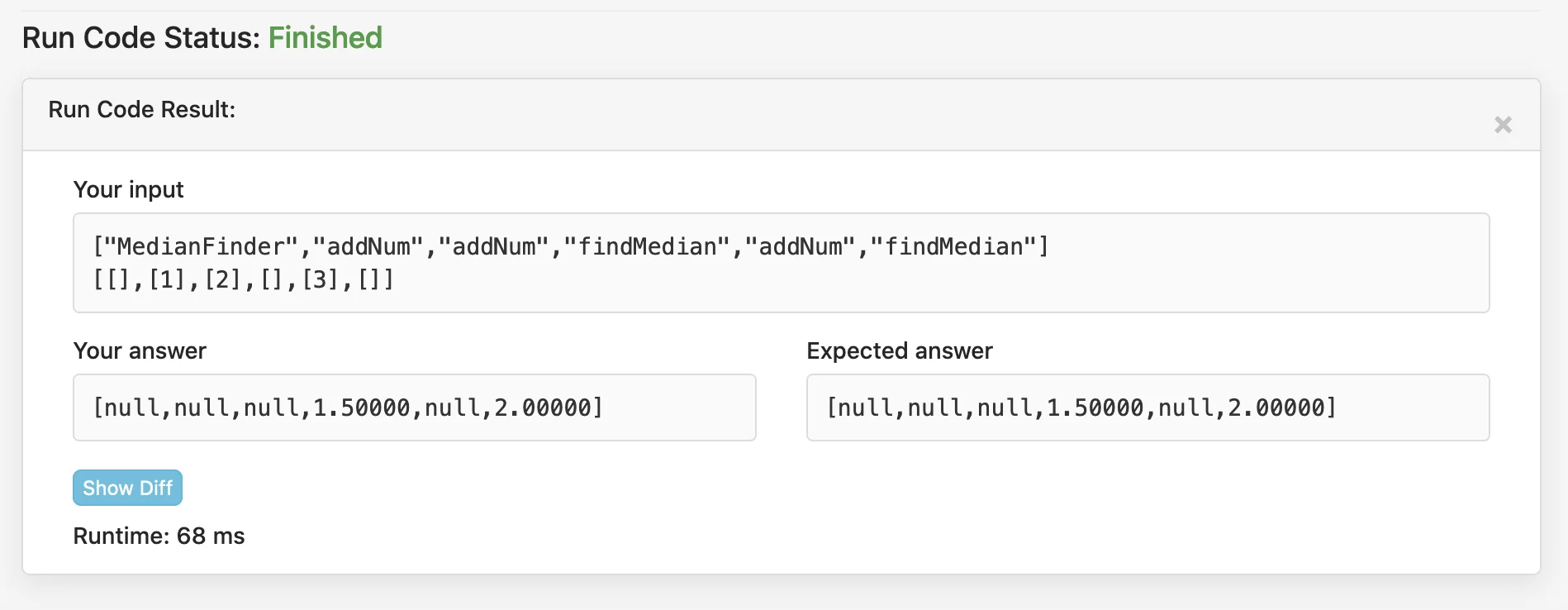Find Median from Data Stream

Thank you

To read more about the technologies, Please subscribe us, You'll get the monthly newsletter having all the published article of the last month.

### OTHER POSTS